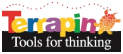Terrapin Resources

# Factorials

Did you ever wonder how many ways to arrange n distinct objects? The answer is the factorial or the product of all positive integers less than or equal to n. For example, if there are three objects, a, b and c they can be arranged in 3 x 2 x 1 = 6 ways. abc, bca, cab, cba, acb, and bac.

These arrangements are called the permutations of the set of objects. Try a permutation of four objects. (You should have 4 x 3 x 2 x1 = 24 arrangements.) Here are variants of a Logo procedure that will tell you the permutations of any number of objects up to about 150. For those of you into graphics, you should be able experimentally check the theory of the math by arranging the permutations of a more modest number of objects from the toolbox.

#### FACTORIAL.lgo

``````TO FACTORING :N
; Limit the number factored to a maximum of 150
LOCAL "COUNTER
MAKE "COUNTER :N
REPEAT :N [MAKE "ANSWER :ANSWER * :COUNTER MAKE "COUNTER :COUNTER - 1]
END

TO FACTORIAL.FOR :N
END

TO FACTORIAL.WHILE :N
LOCAL "I
MAKE "I :N
WHILE [:I > 0] [MAKE "ANSWER :ANSWER * :I MAKE "I :I - 1]
END

TO FACTORIAL.GO :N
LOCAL "I
MAKE "I :N
LABEL "LOOP